StatLect

# Discrete Fourier transform - Spectra

The Discrete Fourier Transform of a vector (or signal) can be used to compute the so-called spectra, which help us to visualize the frequency components of the signal.

In this lecture we define and explain the amplitude, power and phase spectra.## The transform

LetRemember that the Discrete Fourier Transform (DFT) of anvectoris anothervectorwhose entries satisfywhereis the imaginary unit.

We can use the DFT to write the vectoras a linear combination of samples of periodic functions having different frequencies:The coefficients of the linear combination are the entries of the DFT divided by.

The linear combination is called the frequency-domain representation of.

## Amplitude spectrum

The amplitude spectrum is a simple transformation of the DFT.

It is anvectorwhose entries are calculated asIn other words, the amplitude spectrum is the vector that contains the absolute values (or moduli) of the coefficients of the frequency-domain representation of.

It shows which frequencies contribute more to the magnitude of.

As explained in the lecture on the DFT or real signals, ifis real, then the amplitude spectrum is symmetric around the Nyquist frequency.

### Example of amplitude spectrum

Here is an example of an amplitude spectrum.

Letand the entries of the vectorbe defined by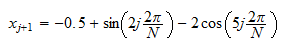As it is customary for spectra, we display the amplitude spectrum ofas a stem plot.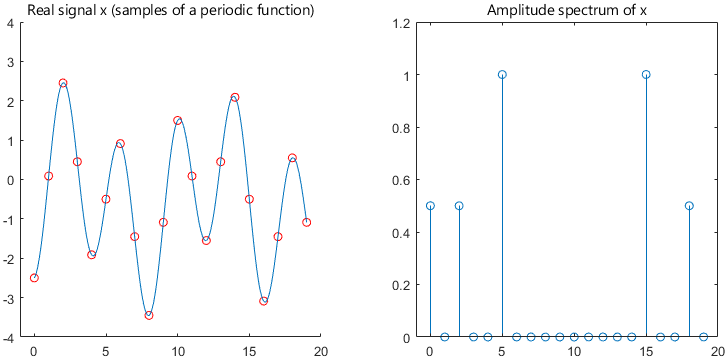As you can see, the spectrum is equal to zero everywhere, except at the frequencies:

•, corresponding to the constant ();

•, corresponding to the term;

•, corresponding to the term;

•and, which are symmetric toand.

The amplitudes are the absolute values of the coefficients of the frequency components (,, -), but the latter two are halved because of the symmetry.

## Power spectrum

The power spectrumis anothervector obtained from the DFT.

Its entries are equal to the squares of the entries of the amplitude spectrum:## Phase spectrum

The phase spectrum shows the phases of the frequency components of.

It is anvectorwhose entries are calculated aswhereandare the real and imaginary parts of.

The functionis the 2-argument arctangent, which returns a value betweenand.

It is the same as the argument of a complex number, that is,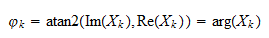Remember thatprovided thatand.

Whenandare both equal to zero (or, equivalently,), the value of(equivalently, of) is undefined. It can be set equal to, as we will do below, to make the phase spectrum easier to read.

### Why is the phase spectrum defined in this way?

To understand why the phase spectrum is defined in this manner, consider a cosine wave:where:

•;

•is the phase;

• the numberof cycles persamples (the frequency) is a positive integer smaller than.

We assume that.

The Discrete Fourier Transform ofis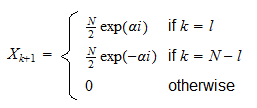This implies that the phase spectrum isProof

We can write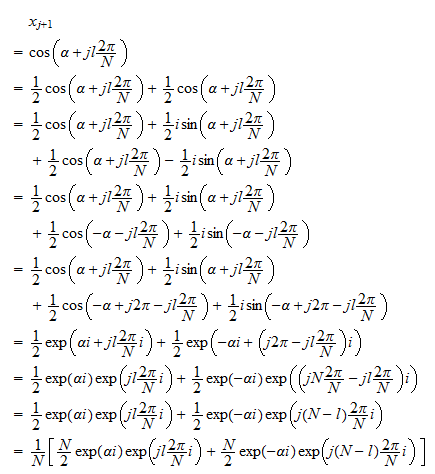The latter expression is the frequency-domain representation ofas a linear combination of the DFT basis functions. Therefore, the coefficients of the linear combination inside the square brackets are the values of the discrete Fourier transform. All the coefficients are equal to, except those corresponding to the frequenciesand, which are equal toandrespectively. Therefore, we have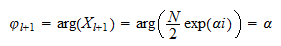and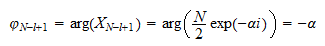### Example of phase spectrum

Here is an example of a phase spectrum.

Letand the entries of the vectorbe defined by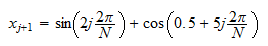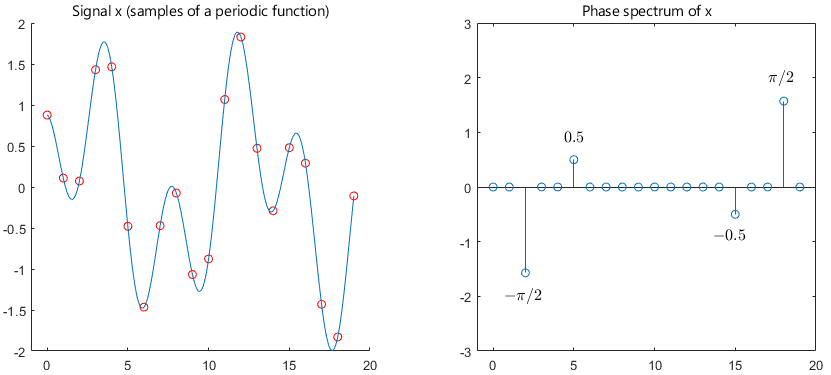The phase spectrum is zero everywhere, except at the following frequencies:

•, where it is equal tobecause;

•, where it is equal to;

•and, which are anti-symmetric toand.